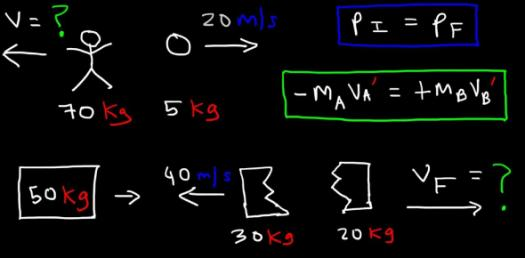# The Law Of Conservation Of Momentum! Physics Trivia Quiz

14 Questions | Attempts: 817
ShareSettings.

• 1.
When comparing the momentum of two moving objects, which of the following is correct?
• A.

The object with the higher velocity will have less momentum if the masses are equal.

• B.

The more massive object will have less momentum if its velocity is greater.

• C.

The less massive object will have less momentum if the velocities are the same.

• D.

The more massive object will have less momentum if the velocities are the same.

• 2.
Two skaters stand facing each other. One skater’s mass is 60 kg, and the other’s mass is 72 kg. If the skaters push away from each other without spinning,
• A.

The lighter skater has less momentum.

• B.

Their momenta are equal but opposite.

• C.

Their total momentum doubles.

• D.

Their total momentum decreases.

• 3.
In a two-body collision,
• A.

Momentum is always conserved.

• B.

Kinetic energy is always conserved.

• C.

Neither momentum nor kinetic energy is conserved.

• D.

Both momentum and kinetic energy are always conserved.

• 4.
A person sitting in a chair with wheels stands up, causing the chair to roll backward across the floor. The momentum of the chair
• A.

Was zero while stationary and increased when the person stood.

• B.

Was greatest while the person sat in the chair.

• C.

Remained the same.

• D.

Was zero when the person got out of the chair and increased while the person sat.

• 5.
Two swimmers relax close together on air mattresses in a pool. One swimmer’s mass is 48 kg, and the other’s mass is 55 kg. If the swimmers push away from each other,
• A.

Their total momentum triples.

• B.

Their momenta are equal but opposite.

• C.

Their total momentum doubles.

• D.

Their total momentum decreases.

• 6.
A 75 kg person walking around a corner bumped into an 80 kg person who was running around the same corner. The momentum of the 80 kg person
• A.

Increased.

• B.

Remained the same.

• C.

Decreased.

• D.

Was conserved.

• 7.
The law of conservation of momentum states that
• A.

The total initial momentum of all objects interacting with one another usually equals the total final momentum.

• B.

The total initial momentum of all objects interacting with one another does not equal the total final momentum.

• C.

The total momentum of all objects interacting with one another is zero.

• D.

The total momentum of all objects interacting with one another remains constant regardless of the nature of the forces between the objects.

• 8.
When two ice skaters initially at rest push off one another, their final momenta are
• A.

Equal in magnitude and direction.

• B.

Equal in magnitude and opposite in direction.

• C.

In the same direction but of different magnitudes

• D.

In opposite directions and possibly of different magnitudes.

• 9.
When two objects interact in an isolated system,
• A.

The momentum of each object is conserved.

• B.

The total momentum of the system is zero.

• C.

The total momentum is conserved only if the objects move in opposite directions.

• D.

The total momentum is always conserved.

• 10.
Conservation of momentum follows from
• A.

Newton’s first law.

• B.

Newton’s second law.

• C.

Newton’s third law.

• D.

The law of conservation of energy.

• 11.
A 6.0 x 10-2 kg tennis ball moves at a velocity of 12 m/s. The ball is struck by a racket, causing it to rebound in the opposite direction at a speed of 18 m/s. What is the change in the ball’s momentum?
• A.

0.36 kg*m/s

• B.

0 kg*m/s

• C.

0.036 kg*m/s

• D.

1.8 kg*m/s

• 12.
A student stumbles backward off a dock and lands in a small boat. The student isn't hurt, but the boat drifts away from the dock with a velocity of 0.85 m/s to the west. If the boat and student each have a mass of 68 kg, what is the student’s initial horizontal velocity?
• A.

0 m/s

• B.

57.8 m/s

• C.

0.85 m/s

• D.

1.7 m/s

• 13.
A 20.0 kg cannonball is fired from a 2.40 x 103 kg. If the cannon recoils with a velocity of 3.5 m/s backwards, what is the velocity of the cannonball?
• A.

420 m/s

• B.

48,000 m/s

• C.

9.81 m/s

• D.

137 m/s

• 14.
A 50.0 g shell fired from a 3.00 kg rifle has a speed of 400.0 m/s. With what speed does the rifle recoil in the opposite direction?
• A.

6.67 m/s

• B.

6666.67 m/s

• C.

21200 m/s

• D.

1220 m/s

## Related TopicsBack to top
×

Wait!
Here's an interesting quiz for you.